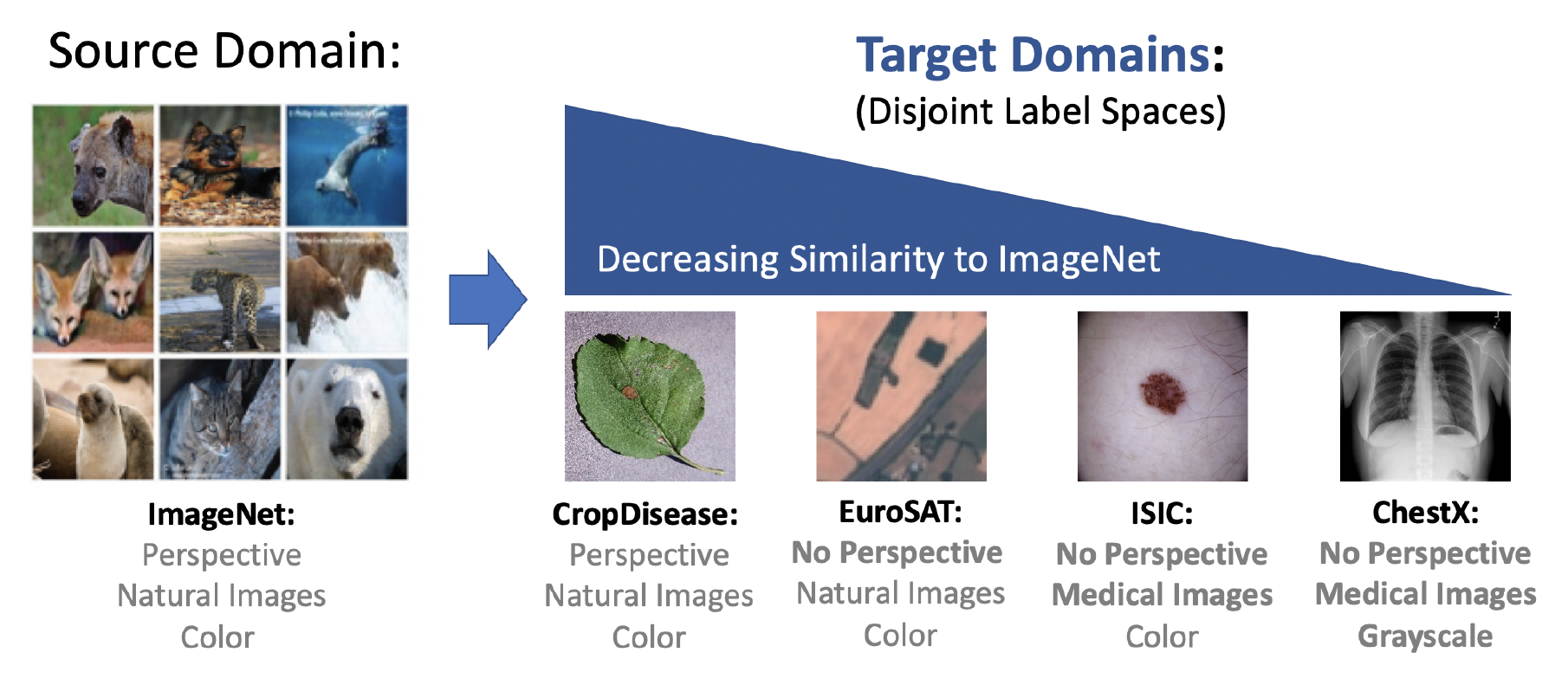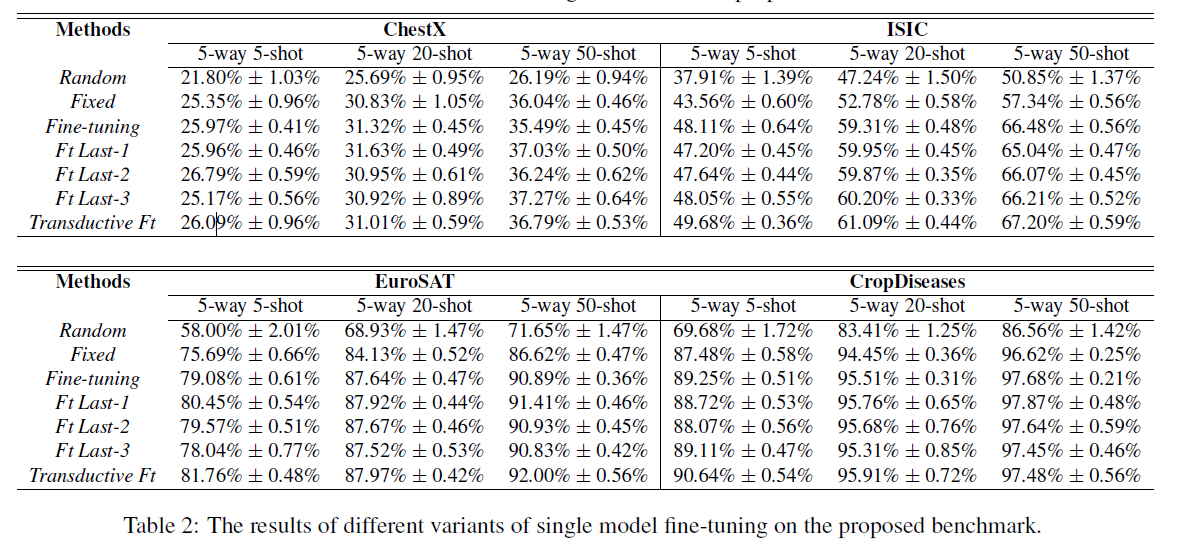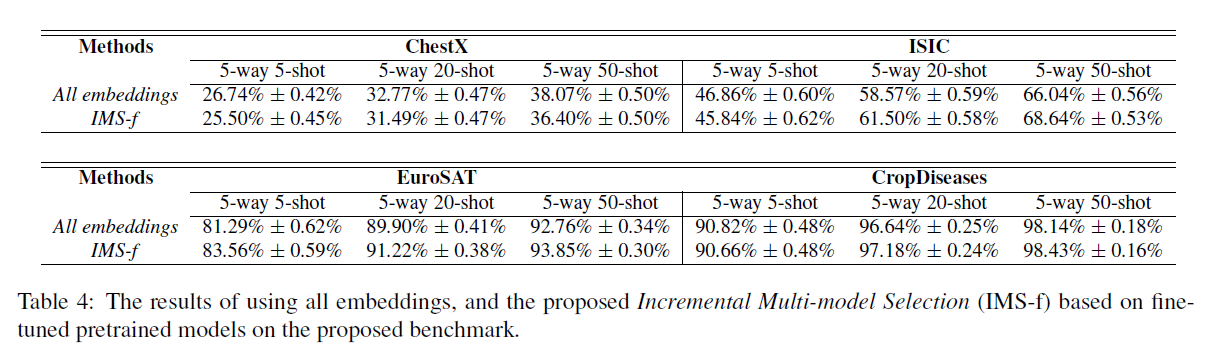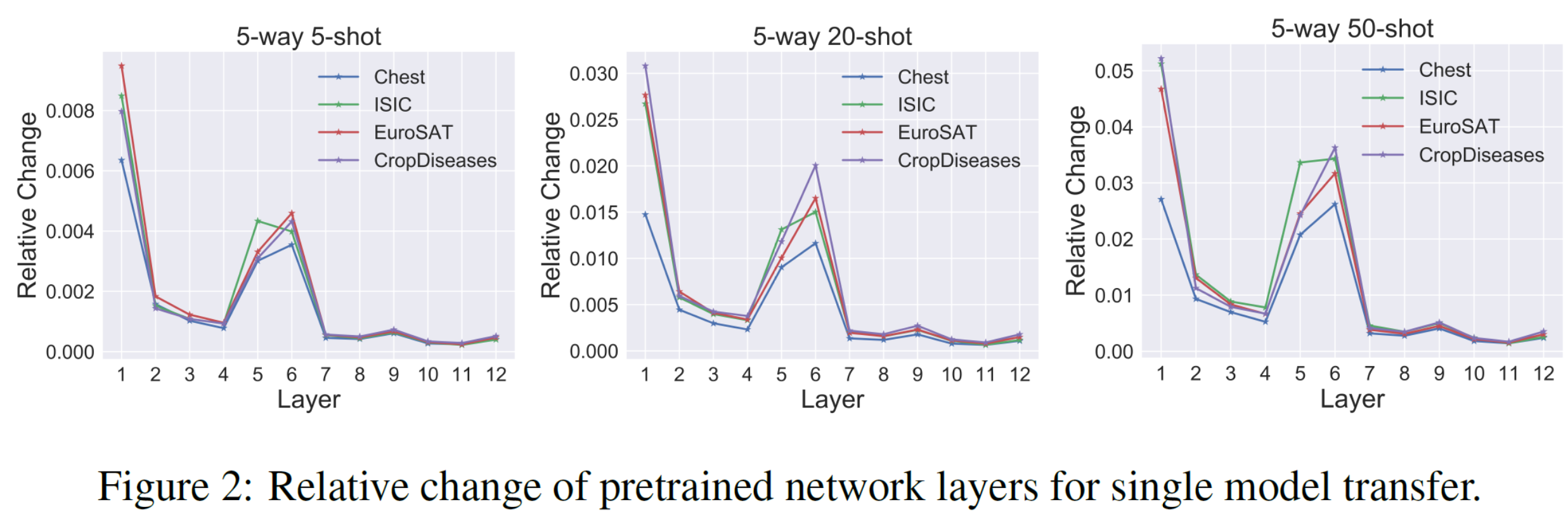Jarvis' Blog (总有美丽的风景让人流连) 总有美丽的风景让人流连

# 跨域少样本学习 (Cross-Domain Few Shot Learning)

2020-05-28
Jarvis
Post

## 1. Introduction• 类别在样本间表现出可预测的变化时
• 前景与背景的对比比较明显时

• 皮肤病诊断
• 放射学诊断 (应该是指医学影像诊断)

### 少样本学习

meta-training vs meta-testing: 类别不重复, 少量的监督样本

Chen等人(A closer look at FSL)1 指出基于 meta-learning 的少样本学习方法在 domain shift 较大的时候表现还不如传统的 pre-training + fine-tuning. 因此本文提出了 跨域少样本学习 (cross-domain few-shot learning, CS-FSL) 并建立起 benchmark, 以 ImageNet 为参照, 本文给出四个类型的数据集:

• 农业: CropDiseases2 彩色, 自然图像, 有自身的特点 (相似度 ❤❤❤❤)
• 卫星图: EuroSAT3 彩色, 自然图像, 无透视变形 (相似度 ❤❤❤)
• 皮肤病: ISIC20184 彩色, 医学影像, 无透视变形 (相似度 ❤❤)
• X光片: ChextX5 灰度, 医学影像, 无透视变形 (相似度 ❤)

## 2. 跨域少样本学习

$\epsilon(f_{\theta})=E_{(x, y)\sim P}[l(f_{\theta}(x), y)].$

base 类的数据从源域采样, novel 类的数据从目标域采样.

• 训练时, 模型在 base 类数据上训练.
• 测试时, 模型在 novel 类数据上测试. 其中会提供支撑集(support set) $$S=\{x_i, y_i\}_{i=1}^{K\times N}$$, 其中包含 $$K$$ 和类别, 每个类别 $$N$$ 个带标签的样本, 称为 K-way N-shot 的少样本学习. 在模型适应到支撑集后使用查询集(query set)测试.

## 3. 研究方法

### 3.1 元学习 Meta-Learning

meta-training 阶段: 模型在 $$T$$ 个 task $$\{\mathcal{T}_i\}_{i=1}^T$$ 上训练, 这 $$T$$ 个 采样于 $$P_s(\mathcal{T})$$ .

meta-testing 阶段: 希望模型可以快速适应于新的 task $$T_j\sim P_t(\mathcal{T})$$ .

### 3.2 迁移学习 Transfer Learning

• 固定 $$f_{\theta}$$ 的参数, 仅用作特征提取器, 在 novel 类上 fine-tune. 但在少样本的情况下及其容易过拟合.

#### 3.2.1 单模型方法

• 固定的特征提取器 (Fixed): 不更新模型
• Fine-tune: 用 novel 类的支撑集更新整个模型
• Fine-tune 最后 k 层 (Ft last-k): 仅用 novel 类的支撑集更新最后 k 层, k 取 1, 2, 3
• Transductive(传导性的) fine-tune (Transductive Ft): novel 类查询图片的 统计值通过 BN 来使用.

• Linear classifier

• Mean-centroid classifier (基于原型6的方法)

• Cosine-similarity based classifier (直接学习原型1) $$c_{i,k}=\frac{f_{\theta}(\hat{x}_i)^T\mathbf{w}_k}{\Vert f_{\theta}(\hat{x}_i)^T\Vert\Vert\mathbf{w}_k\Vert}$$ 其中 $$\mathbf{W}_k$$ 为代表第 k 个类别向量, 可以看作原型, 但是是通过反向传播学出来的.#### 3.2.2 多模型方法 (论文提出的方法)

$\underset{I\subseteq F,\;(x, y)\sim P_t}{\arg\min}l(f_s(T(\{a(x):a \in I\})), y)$

$$I$$ 的筛选方法:

1. 每一个预训练模型的每一层都训练一个分类器, 然后每个模型最好的层加入 $$I_1$$
2. 把 $$I_1$$ 中的层累进式地加入 $$I$$, 可以降低误差则保留, 否则丢弃

## 4. 实验

### 4.1 四个数据集 + 三种训练方法 + 三种分类器

• 元学习• 单模型迁移• 多模型迁移### 4.2 单模型迁移方法, 微调对参数的影响• 第一层的参数变化最大: 说明跨域学习所需要的低级特征是相当不同的
• 有一些层的参数变化很小

• 重置 ambient 层的参数对模型几乎没有影响
• 充值 critical 层的参数让模型几乎失去预测能力(变成随机输出)

### 4.3 多模型迁移方法, 预训练模型的选择性• 不同数据集对预训练模型是有倾向性的
1. A closer look at few-shot classification
Wei-Yu Chen, Yen-Cheng Liu, Zsolt Kira et al.

2. Using deep learning for image-based plant disease detection
Sharada P Mohanty, David P Hughes, and Marcel Salathe
[link]. In Frontiers in plant science, 7:1419, 2016.

3. Eurosat: A novel dataset and deep learning benchmark for land use and land cover classification
Patrick Helber, Benjamin Bischke, Andreas Dengel et al
[link]. In IEEE Journal of Selected Topics in Applied Earth Observations and Remote Sensing, 12(7):2217–2226, 2019.

4. The ham10000 dataset, a large collection of multi-source dermatoscopic images of common pigmented skin lesions
Philipp Tschandl, Cliff Rosendahl, and Harald Kittler
[link]. In Scientific data, 5:180161, 2018.

5. ChestX-Ray8: Hospital-scale chest x-ray database and benchmarks on weakly-supervised classification and localization of common thorax diseases
Xiaosong Wang, Yifan Peng, Le Lu et al